## Getting Started

This presentation is no help if you can’t get the python packages it uses! There are a few options here. If you’re not already set up, probably the easiest way to install python packages is pip. If you don’t already have it, here are installation instructions. I’m a big fan of the anaconda python distrubution, which comes pre-loaded with basically everything I’m using today. Just use whatever works for you.

For example, installing numpy is easy, in a command line:

pip install numpy

If you’re using anaconda, you can also use their package manager:

conda install numpy

Pip is probably the preferred method, even when you’re using anaconda. The packages I’m discussing today are numpy, scipy, pandas, matplotlib, seaborn.

Other useful tools are Numba and Cython. As far as I know, all of these are in both package managers.

I’ve recently been told that pandas depends on cython and seaborn depends on pandas.

You should therefore install cython -> pandas -> seaborn in that order.

This talk was made using a jupyter notebook.

## Python is slow! Why bother?

This is a common reason people want to avoid python, and it’s half true. See for example this function that performs a matrix multiplication:

import math
N = 300

mat1 = [[1.5*i + j for i in range(N)] for j in range(N)]
mat2 = [[2.5*i + j for i in range(N)] for j in range(N)]

def mmult(mat1, mat2):
mat3 = [[0.0 for i in range(N)] for j in range(N)]

for i in range(N):
for k in range(N):
for j in range(N):
mat3[i][k] += mat1[i][j] * mat2[j][k]
return mat3

%time m3 = mmult(mat1, mat2)
CPU times: user 3.78 s, sys: 0 ns, total: 3.78 s
Wall time: 3.78 s

Well that doesn’t seem so bad! This would take longer to do by hand! It actually is pretty slow. Here’s a look at some of the reasons why. It has to do with the fact that python is dynamically typed and interpreted, rather than compiled. Additionally, the arrays in native python aren’t laid out in one chunk of memory.

## It doesn’t have to be this way! (Numpy section)

If you’re going to be doing serious numerical work in python, numpy is essential. If you’re doing a lot of work with arrays, the performance difference between raw python and C/C++/Fortran can easily be several orders of magnitude. Numpy is focused on fast, efficient manipulation of arrays. They can be any size, any dimension as long as your computer can store it. Numpy methods are compiled and very efficient (comparable to compiled code). Let’s take a look at our matrix multiply again:

import numpy as np # convention

npmat1 = np.asarray(mat1) # use numpy arrays, they are contiguous in memory
npmat2 = np.asarray(mat2)

%time npmat3 = np.dot(npmat1, npmat2) # dot is used for dot product or matrix multiply
CPU times: user 4 ms, sys: 4 ms, total: 8 ms
Wall time: 5.86 ms

WHOA! Blazing fast! Morale of the story: if there is a numpy function to do it, use it! And there is a numpy function for a lot of stuff, here’s a few useful ones:

np.linalg.eig - eigenvectors/values

np.zeros - create an array of zeros

np.linspace - create an array of values spaced linearly between 2 endpoints

np.sort - Sort an array

np.amax - Return maximum value of array

np.random.rand - create array of random values in [0, 1)

There’s about 3 trillion more …

## A few useful tips about numpy arrays:

### 1) Most ‘normal’ operations are element-wise:

A = np.array([[1, 2, 3]
,[4, 5, 6]
,[7, 8, 9]])
print(10 + A)
[[11 12 13]
[14 15 16]
[17 18 19]]
print(2 * A)
[[ 2  4  6]
[ 8 10 12]
[14 16 18]]
print(A == 5)
[[False False False]
[False  True False]
[False False False]]
B = np.array([[10, 20, 30]
,[40, 50, 60]
,[70, 80, 90]])
print(A + B)
[[11 22 33]
[44 55 66]
[77 88 99]]

### 2) You can ‘slice’ numpy arrays

print(A, '\n')
print(A[:, 2])  # 3rd column (starts at 0)
[[1 2 3]
[4 5 6]
[7 8 9]]

[3 6 9]
print(A[1, :])  #Second row
[4 5 6]
print(A[0:2, 0:2]) # Top-Left 2x2 section
[[1 2]
[4 5]]

### 3) You can index a numpy array… with a numpy array

indices = np.array([0, 2])
print(A)
[[1 2 3]
[4 5 6]
[7 8 9]]
print(A[indices])  # first and third row
[[1 2 3]
[7 8 9]]
print(A[:,indices])  # first and third column
[[1 3]
[4 6]
[7 9]]
indices2 = np.asarray([[True, True, False]
,[False, True, True]
,[False, False, False]])
print(A, '\n')
print(A[indices2])  # All elements where indices2 is True
[[1 2 3]
[4 5 6]
[7 8 9]]

[1 2 5 6]

### 4) And you can combine operations

symmetric = np.array([[1, 4, 4]
,[4, 1, 4]
,[4, 4, 1]])

asymmetric = np.array([[1, 4, 4]
,[7, 1, 4]
,[42, 5, 1]])

Maybe to check if a matrix is symmetric

np.all(symmetric.T == symmetric)
True
np.all(asymmetric.T == asymmetric)  # WARNING if using floats, compare
# with np.isclose(x.T, x)
False

Or to sort eigenvales and eigenvectors so that the eigenvalues are in ascending order, and you keep track of the vectors!

vals, vecs = np.linalg.eig(symmetric)
print(vals)
print(vecs)
print('\n')

indices_of_sorted_vals = np.argsort(vals)
print(vals[indices_of_sorted_vals])
print(vecs[:, indices_of_sorted_vals])  # columns are rearranged
[-3.  9. -3.]
[[-0.81649658  0.57735027  0.19219669]
[ 0.40824829  0.57735027 -0.7833358 ]
[ 0.40824829  0.57735027  0.59113912]]

[-3. -3.  9.]
[[-0.81649658  0.19219669  0.57735027]
[ 0.40824829 -0.7833358   0.57735027]
[ 0.40824829  0.59113912  0.57735027]]

## Scipy

We’re all scientists here, so It would be silly to avoid scipy. It’s a library of scientifically relevant tools. Here’s a list of some of the submodules:

Special functions (scipy.special)

Integration (scipy.integrate)

Optimization (scipy.optimize)

Interpolation (scipy.interpolate)

Fourier Transforms (scipy.fftpack)

Signal Processing (scipy.signal)

Linear Algebra (scipy.linalg)

Sparse Eigenvalue Problems with ARPACK

Compressed Sparse Graph Routines (scipy.sparse.csgraph)

Spatial data structures and algorithms (scipy.spatial)

Statistics (scipy.stats)

Multidimensional image processing (scipy.ndimage)

File IO (scipy.io)

Weave (scipy.weave)

The various python modules make it super easy to do lots of things, like making a function that returns the nth harmonic oscillator wavefunction:

from math import factorial, pi
from scipy.special import hermite as h

def psi(x, n):
N = 1.0 / np.sqrt(float(2**n) * factorial(n)) * (1.0 / pi)
return N * np.exp(-x*x / 2.0) * h(n)(x)

If I try to cover all of scipy it will get boring fast… you can look up functions as you need them

## Matplotlib

Matplotlib is the tool to go to for quick plotting stuff in Python. It can handle 2D, 3D, and even animations.

import matplotlib.pyplot as plt
# Jupyter  "Magic", just so you see the images in the notebook
%matplotlib inline

fig = plt.figure(figsize=(6,4))

x = np.linspace(0, 10, 100)   # list of x from 0 to 10 by 100 steps
y = np.sin(x)                 # take the sign of each element in x
plt.plot(x, y)                # do I have to explain this one?
plt.show()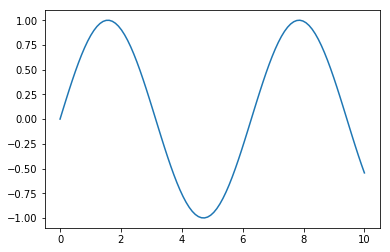To plot multiple things at once, call plt.plot() more than once

def plot_HO(N): # plot N wavefunctions
fig = plt.figure(figsize=(6,6))

ymax = N + 1.5
xmax = math.sqrt(ymax)    # figuring out plot ranges
x = np.linspace(-xmax, xmax, 100)

for n in range(N):                       # from n = [0...N)
plt.plot(x, psi(x, n) + (n + 0.5))   # Plot the wavefunction offset by
# energy

plt.plot(x, 0.5 * x*x, 'k')  # Plot the potential

plt.xlim(-xmax, xmax)
plt.ylim(0, ymax)
plt.show()
plot_HO(5)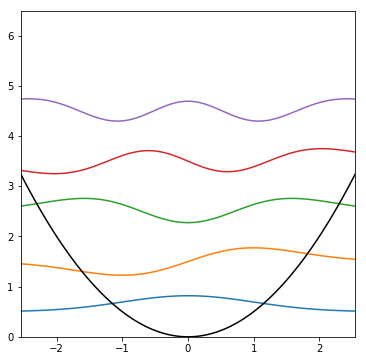Matplotlib has functions for scatterplots, pie charts, bar graphs, heatmaps blah blah blah…

## Pandas

I recently started using Pandas in my research, and It’s already proven incredibly useful for data analysis. The library is focused on the Series and the DataFrame.

import pandas as pd

series1 = pd.Series([4, 2.5, 8, 72])
series1
0     4.0
1     2.5
2     8.0
3    72.0
dtype: float64

I don’t know about you, but this isn’t the most impressive thing to me… just hold on.

Here’s an example of a DataFrame, the reason I’m in love with this library

Cols = ['Rain', 'Temperature', 'Wind']
Dates = pd.date_range('20161020', periods=10)
data = np.random.rand(10, 3) # 3 cols 10 rows

df= pd.DataFrame(data, index=Dates, columns=Cols)
df
Rain Temperature Wind
2016-10-20 0.193955 0.350702 0.618494
2016-10-21 0.707791 0.823698 0.548552
2016-10-22 0.851024 0.983293 0.709565
2016-10-23 0.434959 0.669047 0.405353
2016-10-24 0.398242 0.252898 0.604027
2016-10-25 0.620698 0.142928 0.551496
2016-10-26 0.438231 0.620494 0.525627
2016-10-27 0.424516 0.189178 0.570212
2016-10-28 0.093206 0.278414 0.980758
2016-10-29 0.429858 0.857150 0.886912
df['Rain']  # Grab all the stuff in 'Rain' column
2016-10-20    0.193955
2016-10-21    0.707791
2016-10-22    0.851024
2016-10-23    0.434959
2016-10-24    0.398242
2016-10-25    0.620698
2016-10-26    0.438231
2016-10-27    0.424516
2016-10-28    0.093206
2016-10-29    0.429858
Freq: D, Name: Rain, dtype: float64
df.loc['2016-10-23']  # locate this date
Rain           0.434959
Temperature    0.669047
Wind           0.405353
Name: 2016-10-23 00:00:00, dtype: float64
df.iloc  # grab the 3rd row
Rain           0.851024
Temperature    0.983293
Wind           0.709565
Name: 2016-10-22 00:00:00, dtype: float64
df.plot()  # Plot all the stuff
<matplotlib.axes._subplots.AxesSubplot at 0x7f67de0d2e80>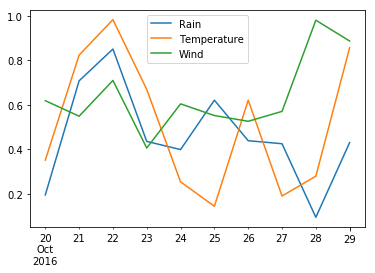df.plot.scatter(x='Rain', y='Temperature')
plt.xlim(0,1)
plt.show()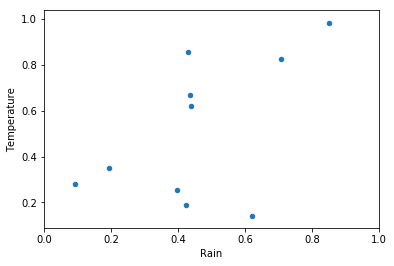You can find data by name and apply an operation to it, like checking which days had small amounts of rain:

df['Rain'] < 0.1
2016-10-20    False
2016-10-21    False
2016-10-22    False
2016-10-23    False
2016-10-24    False
2016-10-25    False
2016-10-26    False
2016-10-27    False
2016-10-28     True
2016-10-29    False
Freq: D, Name: Rain, dtype: bool

Hmm, that’s pretty nice isn’t it? What if we could easily select only the parts that satisfy our condition? ….

df[df['Rain'] < 0.1]
Rain Temperature Wind
2016-10-28 0.093206 0.278414 0.980758

Ok that’s pretty neat. I think this alone is useful. But we’re just getting started!

What if we could find all parts of our data frame that satisfy a given condition, then plot two other columns of that data? Say no more…

TempsAbove = df[df['Temperature'] > 0.5]
TempsAbove.plot.scatter('Rain', 'Wind')
plt.show()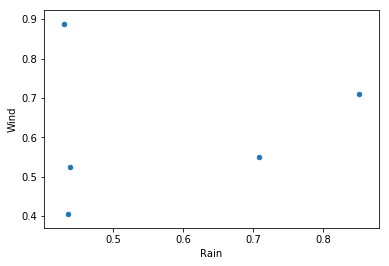The reason I like this library so much is that you can use the past few examples, combining them as you wish, and analyze huge amounts of data in seconds. Lately I’ve written a function to do a custom plot on each row of a dataframe (optionally you can apply a condition to a dataframe and pass that through). I take my output files, parse them and put them into a dataframe. This lets me go from a directory of output files to a ton of plots in seconds, with little effort.

## Seaborn

*Update: As of matplotlib 2.0, the style defaults there are actually good. Seaborn is now less useful if you’re not doing statistical plots. *

Let’s face it: I’ve been to enough talks to know that most people don’t know how to make visually appealing figures. Well the good news is that you don’t have to. Trust in Seaborn, for it is magnificent.

import seaborn as sns # importing changes defaults
plt.ylim(-1.1, 1.1)
plt.plot(x, y)
[<matplotlib.lines.Line2D at 0x7f67ddf5d3c8>]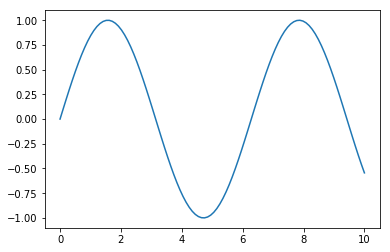This is the default with nothing done, the library was made to have nice colorschemes out of the box, but I prefer a less intrusive background

sns.set_style('white')
plt.ylim(-1.1, 1.1)
plt.plot(x, y)
[<matplotlib.lines.Line2D at 0x7f67dafe6cf8>]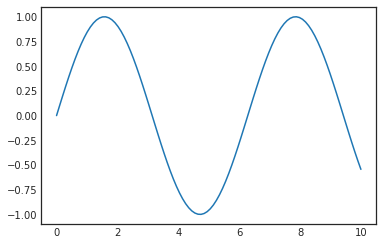Remember our harmonic oscillator plots?

plot_HO(5)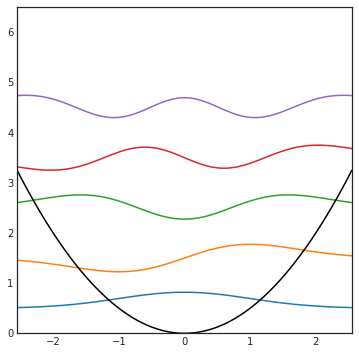There are a lot of built in plots, mostly for discrete data points and statistics. I mostly use the color palettes and things like that, but if you need to do statistical plots, look no further

Cols = ['Rain', 'Temperature', 'Wind']
N = 100
Dates = pd.date_range('20161020', periods=N)

from scipy.signal import gaussian
rain = gaussian(N, 20)
Temp = np.cos(np.linspace(0, 6.28, N))
Wind = np.cos(np.arange(N)) * 0.3
data = np.zeros((N,3))
data[:, 0] = rain
data[:, 1] = Temp
data[:, 2] = Wind

df2 = pd.DataFrame(data, index=Dates, columns=Cols)
df2.plot()
plt.ylim(-1.1, 1.1)
plt.show()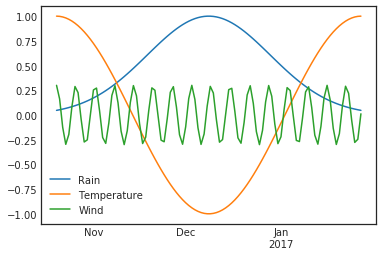sns.jointplot(x='Rain', y='Wind', data=df2)
<seaborn.axisgrid.JointGrid at 0x7f67ddffb320>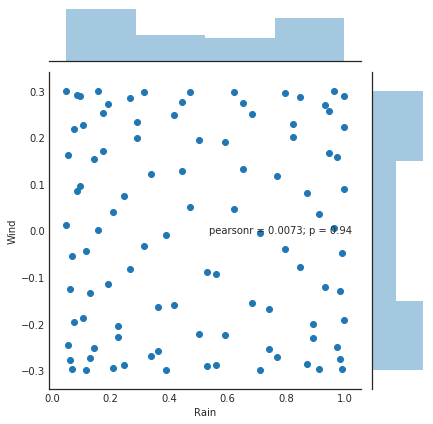cols = ['Abs', 'Conc', 'Molecule', 'Temp']

Temps = [0, 25, 50, 75]
Molecules = ['Blue Dye', 'Orange Dye']
Absorptivity = [0.2, 0.1]   # whatever units makes this reasonable
Concs = [0.1, 0.5, 0.8, 1.2]

data = []
for temp in Temps:
for j in range(len(Molecules)):
for conc in Concs:
A = Absorptivity[j] * conc * np.exp((273 + temp) / 300)
A += np.random.rand() * 0.05
data.append([A, conc, Molecules[j], temp])

AbsDF = pd.DataFrame(data, columns=cols)
fig = plt.figure(figsize=(5, 5))
sns.lmplot(x='Conc', y='Abs', hue='Molecule',
col='Temp', data=AbsDF, col_wrap=2)
plt.show()
<matplotlib.figure.Figure at 0x7f67d947c198>